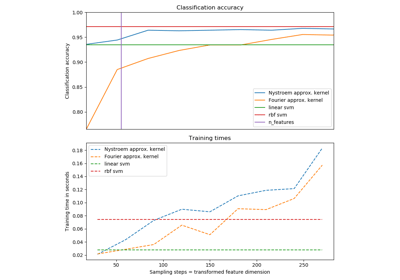/scikit-learn

# sklearn.kernel_approximation.RBFSampler

`class sklearn.kernel_approximation.RBFSampler(gamma=1.0, n_components=100, random_state=None)` [source]

Approximates feature map of an RBF kernel by Monte Carlo approximation of its Fourier transform.

It implements a variant of Random Kitchen Sinks.

Read more in the User Guide.

Parameters: `gamma : float` Parameter of RBF kernel: exp(-gamma * x^2) `n_components : int` Number of Monte Carlo samples per original feature. Equals the dimensionality of the computed feature space. `random_state : int, RandomState instance or None, optional (default=None)` If int, random_state is the seed used by the random number generator; If RandomState instance, random_state is the random number generator; If None, the random number generator is the RandomState instance used by `np.random`.

#### Notes

See “Random Features for Large-Scale Kernel Machines” by A. Rahimi and Benjamin Recht.

 “Weighted Sums of Random Kitchen Sinks: Replacing minimization with randomization in learning” by A. Rahimi and Benjamin Recht. (http://people.eecs.berkeley.edu/~brecht/papers/08.rah.rec.nips.pdf)

#### Examples

```>>> from sklearn.kernel_approximation import RBFSampler
>>> from sklearn.linear_model import SGDClassifier
>>> X = [[0, 0], [1, 1], [1, 0], [0, 1]]
>>> y = [0, 0, 1, 1]
>>> rbf_feature = RBFSampler(gamma=1, random_state=1)
>>> X_features = rbf_feature.fit_transform(X)
>>> clf = SGDClassifier(max_iter=5)
>>> clf.fit(X_features, y)
...
SGDClassifier(alpha=0.0001, average=False, class_weight=None,
early_stopping=False, epsilon=0.1, eta0=0.0, fit_intercept=True,
l1_ratio=0.15, learning_rate='optimal', loss='hinge', max_iter=5,
n_iter=None, n_iter_no_change=5, n_jobs=None, penalty='l2',
power_t=0.5, random_state=None, shuffle=True, tol=None,
validation_fraction=0.1, verbose=0, warm_start=False)
>>> clf.score(X_features, y)
1.0
```

#### Methods

 `fit`(X[, y]) Fit the model with X. `fit_transform`(X[, y]) Fit to data, then transform it. `get_params`([deep]) Get parameters for this estimator. `set_params`(**params) Set the parameters of this estimator. `transform`(X) Apply the approximate feature map to X.
`__init__(gamma=1.0, n_components=100, random_state=None)` [source]
`fit(X, y=None)` [source]

Fit the model with X.

Samples random projection according to n_features.

Parameters: `X : {array-like, sparse matrix}, shape (n_samples, n_features)` Training data, where n_samples in the number of samples and n_features is the number of features. `self : object` Returns the transformer.
`fit_transform(X, y=None, **fit_params)` [source]

Fit to data, then transform it.

Fits transformer to X and y with optional parameters fit_params and returns a transformed version of X.

Parameters: `X : numpy array of shape [n_samples, n_features]` Training set. `y : numpy array of shape [n_samples]` Target values. `X_new : numpy array of shape [n_samples, n_features_new]` Transformed array.
`get_params(deep=True)` [source]

Get parameters for this estimator.

Parameters: `deep : boolean, optional` If True, will return the parameters for this estimator and contained subobjects that are estimators. `params : mapping of string to any` Parameter names mapped to their values.
`set_params(**params)` [source]

Set the parameters of this estimator.

The method works on simple estimators as well as on nested objects (such as pipelines). The latter have parameters of the form `<component>__<parameter>` so that it’s possible to update each component of a nested object.

Returns: self
`transform(X)` [source]

Apply the approximate feature map to X.

Parameters: `X : {array-like, sparse matrix}, shape (n_samples, n_features)` New data, where n_samples in the number of samples and n_features is the number of features. `X_new : array-like, shape (n_samples, n_components)`

## Examples using `sklearn.kernel_approximation.RBFSampler`Explicit feature map approximation for RBF kernels